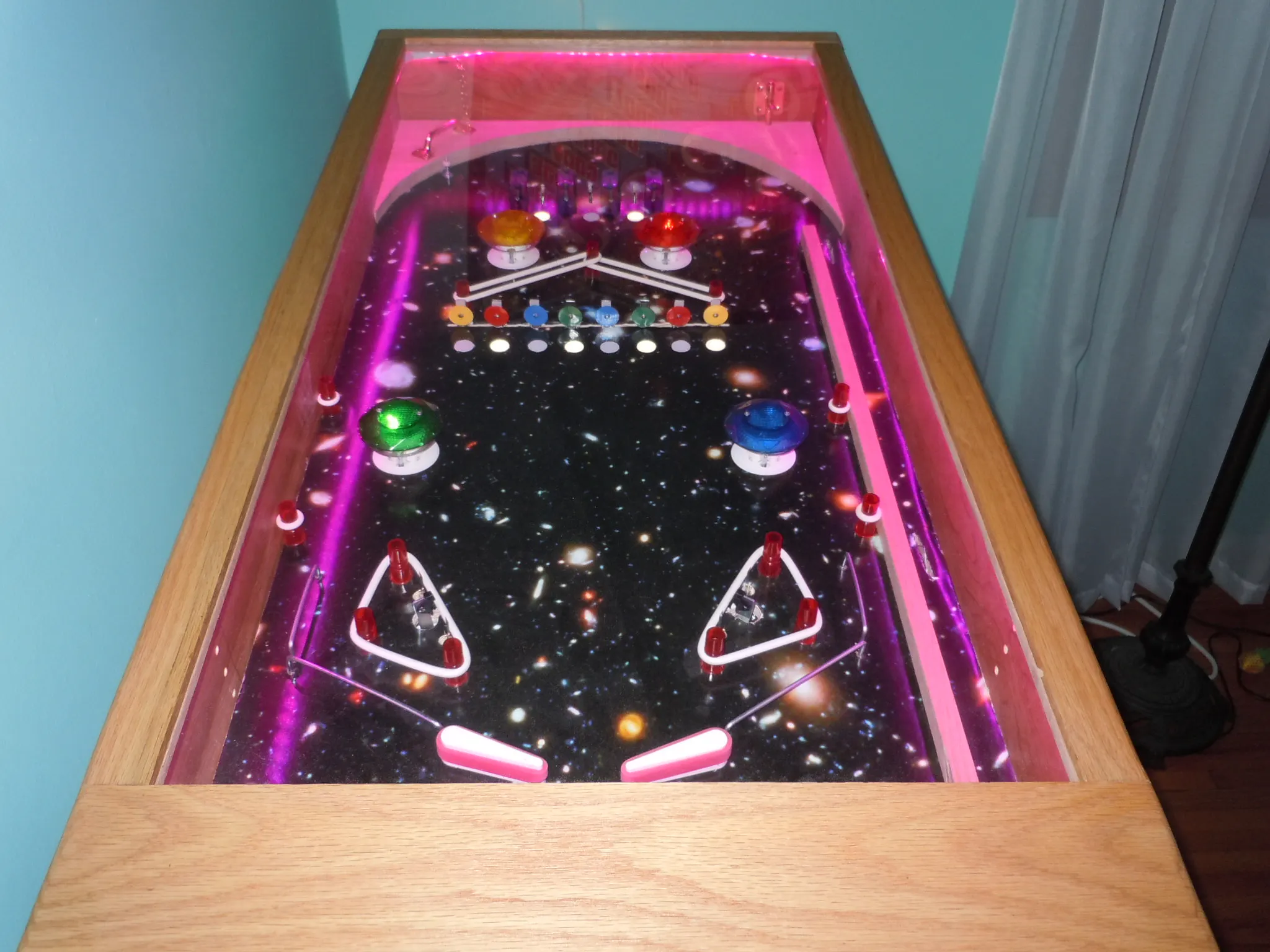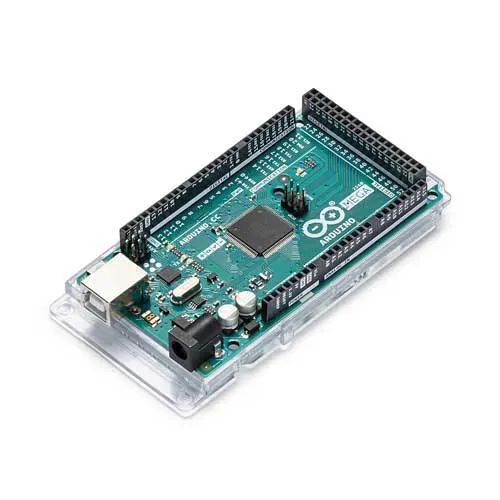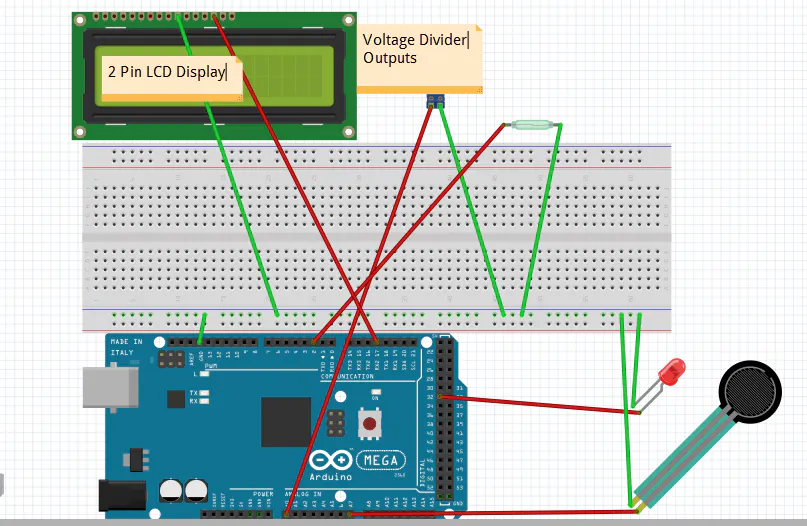# Arduino Controlled Pinball Machine

A standard sized pinball machine made using parts from the home store and pinball supply houses. The game play is controlled by an Arduino.

ExpertFull instructions provided65,244## Things used in this project

### Hardware componentsArduino Mega 2560
×1
×1
 24V Power Supply
×1
×1
 Pop Bumper Assembly
×1
 Pop Bumper Switch
×1
 Target Switch
×1
 Rollover Switch
×1
 Flipper Assembly
×1
 LED Lamps
×1

## Schematics

### Basic Component Schematic of the Arduino Pinball Machine## Code

### Arduino Pinball Machine Code

Arduino
Allows the Arduino to control the game play of a home made pinball machine
```  const int TxPin = 17;
long Score = 0;
long OldScore = 0;
long Target = 1;
long Pop = 1;
long Roll = 10;
int Targets;
int Rolls;
int Pops;
int Milli = 10;
int Sum = 0;
int Flash = 100;
int Ball = 0;
int i=0;
int Shot = 0;
int Lost = 0;
int Pressure = 1024;

#include <SoftwareSerial.h>;
SoftwareSerial mySerial = SoftwareSerial(255, TxPin);

void setup() {
/* Words without an s are the value achieved by interacting with a device.
* Works with an s keep track of which individual ones were interacted with.
* The latter is needed to determine when all have been hit and the value needs upgrading
* and the lights need turning off.
*/
pinMode(TxPin, OUTPUT);
digitalWrite(TxPin, HIGH);
mySerial.begin(9600);
mySerial.write(12);                 // Clear
mySerial.write(17);                 // Turn backlight on

//target inputs
pinMode(2,INPUT_PULLUP);
pinMode(3,INPUT_PULLUP);
pinMode(4,INPUT_PULLUP);
pinMode(5,INPUT_PULLUP);
pinMode(6,INPUT_PULLUP);
pinMode(7,INPUT_PULLUP);
pinMode(8,INPUT_PULLUP);
pinMode(9,INPUT_PULLUP);
//rollover inputs
pinMode(10,INPUT_PULLUP);
pinMode(11,INPUT_PULLUP);
pinMode(12,INPUT_PULLUP);
//lower ball shot switch
pinMode(15,INPUT_PULLUP);
//upper ball shot switch
pinMode(16,INPUT_PULLUP);
//lcd output
pinMode(17,OUTPUT);
//target lights, respective
pinMode(32,OUTPUT);
pinMode(33,OUTPUT);
pinMode(34,OUTPUT);
pinMode(35,OUTPUT);
pinMode(36,OUTPUT);
pinMode(37,OUTPUT);
pinMode(38,OUTPUT);
pinMode(39,OUTPUT);
//rollover lights, respective
pinMode(40,OUTPUT);
pinMode(41,OUTPUT);
pinMode(42,OUTPUT);
//pop bumper lights
pinMode(50,OUTPUT);
pinMode(51,OUTPUT);
pinMode(52,OUTPUT);
pinMode(53,OUTPUT);
}

void loop() {
// put your main code here, to run repeatedly:
//If a pull-down resistor is used, the input pin will be LOW when the switch is open and HIGH when the switch is closed.
//check if a target was hit

//****** Targets *****

for (int i=0; i<8; i++){
//Target activated
Targets[i]=1;
Score = Score + Target;
//turn on Target light
digitalWrite(i+32,HIGH);
//delay so as not get multiple points for one hit
delay(Milli);
break;
}
}
Sum = 0;
for (int i=0; i<8; i++){
Sum = Sum + Targets[i];
}
if (Sum == 8){
//all Targets lit, so flash and then turn off.
for (int j=0; j<3; j++){
for (int i=0; i<8; i++){
digitalWrite(i+32, LOW);
}
delay(Flash);
for (int i=0; i<8; i++){
digitalWrite(i+32, HIGH);
}
delay(Flash);
}
for (int i=0; i<8; i++){
digitalWrite(i+32, LOW);
Targets[i]=0;
}
delay(Flash);
//Multiply target value by 10
Target = Target * 5;
//goto Skip;
}

// ***********  Rollovers *********

for (int i=0; i<3; i++){
//rollover activated
Rolls[i]=1;
Score = Score + Roll;
//turn on rollover light
digitalWrite(i+40,HIGH);
//delay so as not get multiple points for one hit
delay(Milli);
break;
}
}
Sum = 0;
for (int i=0; i<3; i++){
Sum = Sum + Rolls[i];
}
if (Sum == 3){
//all rollovers lit, so flash and then turn off.
for (int j=0; j<3; j++){
for (int i=0; i<3; i++){
digitalWrite(i+40, LOW);
}
delay(Flash);
for (int i=0; i<3; i++){
digitalWrite(i+40, HIGH);
}
delay(Flash);
}
for (int i=0; i<3; i++){
digitalWrite(i+40, LOW);
Rolls[i]=0;
}
delay(Flash);
//Multiply score by 2
Score = Score * 2;
Roll = Roll * 10;
//goto Skip;
}

//**********  Pop Bumpers **********

for (int i=0; i<4; i++){
//pop activated
Pops[i]=1;
Score = Score + Pop;
//turn on pop bumper light
digitalWrite(i+50,HIGH);
//delay so as not get multiple points for one hit
//mySerial.print(" ");
delay(Milli);
break;
}
}
Sum = 0;
for (int i=0; i<4; i++){
Sum = Sum + Pops[i];
}
if (Sum == 4){
//all pop bumpers lit, so flash and then turn off.
for (int j=0; j<3; j++){
for (int i=0; i<4; i++){
digitalWrite(i+50, LOW);
}
delay(Flash);
for (int i=0; i<4; i++){
digitalWrite(i+50, HIGH);
}
delay(Flash);
}
for (int i=0; i<4; i++){
digitalWrite(i+50, LOW);
Pops[i]=0;
}
delay(Flash);
//Multiply target value by 10
Pop = Pop * 2;
//goto Skip;
}
Skip:

//Determine ball number
//ball hit lower alley switch
//if not already done so, increase Ball
if (Shot == 0){
//Set Lost = 0 since not on pressure pad
Lost = 0;
//set OldScore so as to reprint ball value on LCD
OldScore =-1;
Ball = Ball + 1;
if (Ball == 6){
Ball = 1;
Score = 0;
Target = 1;
Roll = 1;
Pop = 1;
}
Shot = 1;
}
}
//ball hit lower alley switch
//if not already done so, increase Ball
if (Shot == 0){
//Set Lost = 0 since not on pressure pad
Lost = 0;
//set OldScore so as to reprint ball value on LCD
OldScore =-1;
Ball = Ball + 1;
if (Ball == 6){
Ball = 1;
Score = 0;
Target = 1;
Roll = 1;
Pop = 1;
}
Shot = 1;
}
}

Shot = 0;
if (Lost == 0){
//Score = Score + 100;
Lost = 1;
if (Ball == 5){
//Game Over
//flash rollovers and then turn off.
for (int j=0; j<3; j++){
for (int i=0; i<3; i++){
digitalWrite(i+40, LOW);
}
delay(Flash);
for (int i=0; i<3; i++){
digitalWrite(i+40, HIGH);
}
delay(Flash);
}
for (int i=0; i<3; i++){
digitalWrite(i+40, LOW);
Rolls[i]=0;
}
// flash pop bumpers and then turn off
for (int j=0; j<3; j++){
for (int i=0; i<4; i++){
digitalWrite(i+50, LOW);
}
delay(Flash);
for (int i=0; i<4; i++){
digitalWrite(i+50, HIGH);
}
delay(Flash);
}
for (int i=0; i<4; i++){
digitalWrite(i+50, LOW);
Pops[i]=0;
}
//Flash Targets and then turn off.
for (int j=0; j<3; j++){
for (int i=0; i<8; i++){
digitalWrite(i+32, LOW);
}
delay(Flash);
for (int i=0; i<8; i++){
digitalWrite(i+32, HIGH);
}
delay(Flash);
}
for (int i=0; i<8; i++){
digitalWrite(i+32, LOW);
Targets[i]=0;
}
mySerial.write(12);                 // Clear
delay(5);
// Required delay
mySerial.print(Score);  // First line
mySerial.write(13);                 // Form feed
mySerial.print("Game Over!!!");   // Second line
}
}
}
//print to LCD
if (Score != OldScore){
mySerial.write(12);                 // Clear
delay(5);                           // Required delay
mySerial.print(Score);  // First line
mySerial.write(13);                 // Form feed
mySerial.print("Ball = ");   // Second line
mySerial.print(Ball);
OldScore = Score;
}
}
```

## Credits

### Bob Blomquist

3 projects • 31 followers
Chemist with computer programming skills.